# Homotopy groups need not determine homology groups

Jump to: navigation, search

## Statement

It is possible to have two path-connected spaces$M_1$ and$M_2$ (in fact, we can choose both$M_1$ and$M_2$ to be compact connected manifolds) such that:

• For every positive integer$k$, there is an isomorphism of groups$\pi_k(M_1) \cong \pi_k(M_2)$ between the Homotopy group (?)s.
• There exists a positive integer$k$ such that the Homology group (?)s$H_k(M_1)$ and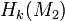$H_k(M_2)$ are not isomorphic.

In particular, because Weak homotopy-equivalent topological spaces (?) have isomorphic homology groups, this gives an example of spaces that have isomorphic homotopy groups but are not weak homotopy-equivalent.

## Facts used

1. Covering map induces isomorphisms on higher homotopy groups

## Proof

Consider the spaces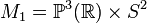$M_1 = \mathbb{P}^3(\R) \times S^2$ and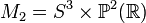$M_2 = S^3 \times \mathbb{P}^2(\R)$. We note that:

• Both the spaces have universal cover$S^3 \times S^2$ (product of 3-sphere and 2-sphere) which is a double cover, so they both have fundamental group$\pi_1(M_1) \cong \pi_1(M_2) \cong \mathbb{Z}/2\mathbb{Z}$.
• By fact (1), the covering maps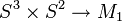$S^3 \times S^2 \to M_1$ and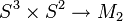$S^3 \times S^2 \to M_2$ both induce isomorphisms on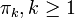$\pi_k, k \ge 1$. Thus, we get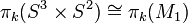$\pi_k(S^3 \times S^2) \cong \pi_k(M_1)$ and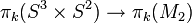$\pi_k(S^3 \times S^2) \to \pi_k(M_2)$ and we thus get$\pi_k(M_1) \cong \pi_k(M_2)$.

Thus, we see that all the homotopy groups match.

However, the homology groups do not. One simple way of seeing this is to note that$M_1$ is orientable, and therefore has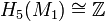$H_5(M_1) \cong \mathbb{Z}$, whereas$M_2$ is non-orientable, so$H_5(M_2) = 0$.

The full homology descriptions are below: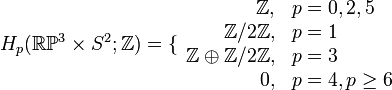$H_p(\R\mathbb{P}^3 \times S^2; \mathbb{Z}) = \lbrace \begin{array}{rl} \mathbb{Z}, & p = 0,2,5\\ \mathbb{Z}/2\mathbb{Z}, & p = 1 \\ \mathbb{Z} \oplus \mathbb{Z}/2\mathbb{Z}, & p = 3\\ 0, & p = 4, p \ge 6 \\\end{array}$

and:$H_p(S^3 \times \R\mathbb{P}^2; \mathbb{Z}) = \lbrace\begin{array}{rl} \mathbb{Z}, & p = 0,3 \\ \mathbb{Z}/2\mathbb{Z}, & p = 1,4 \\ 0, & p = 2, p \ge 5 \\\end{array}$